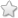# TNSB - 8 - Maths :: Geometry - Term 3

## Course Content / Subtopic list:

1. Introduction, Median Of A Triangle, Centroid, Properties Of The Centroid Of A Triangle,
2. Altitude Of A Triangle, Perpendicular Bisector,Circumcentre, Angle Bisector, Incentre
3. Construction Of Certain Quadrilaterals, Construction Of A Rhombus- Construction Of A Rhombus When One Side And One Diagonal Are Given,Construction Of A Rhombus When One Side And One Angle Are Given:
4. Construction Of A Rhombus When Two Diagonals Are Given, Construction Of A Rhombus When One Diagonal And One Angle Are Given, Construction Of A Rectangle When Its Length And Breadth Are Given:
5. Construction Of A Rectangle When Length And Breadth Are Given, Construction Of A Square When Its Side Is Given, Construction Of A Square When Its Diagonal Is Given:

## Worksheets

Worksheets [Version 2021]

Blank

Worksheets [Version 2021](0 votes)